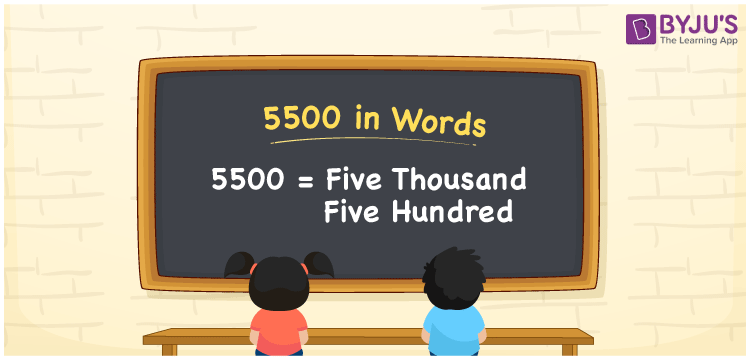# 5500 in Words

We can express 5500 in words as Five Thousand Five Hundred. It can also be written in words as Fifty-Five Hundred. If you have purchased a silk saree worth rupees 5500, you can say, “ I have bought a silk saree worth Rs 5500”. We know that we can convert 5500 to words using the place value chart. Also, we know that 5500 is a cardinal number since it indicates a specific quantity.

 5500 in words Five Thousand Five Hundred Five Thousand Five Hundred in numerical form 5500

## 5500 in English Words

Usually we write numbers in words using the English alphabet. Hence, we can read 5500 in English as “Five Thousand Five Hundred”.## How to Write 5500 in Words?

Here, let us glance at the conversion of 5500 to words using the place value chart. The number 5500 has 4 digits. So, let’s make a chart that represents the place value up to 4 digits

 Thousands Hundreds Tens Ones 5 5 0 0

Therefore, we can write the expanded form as:

5 × Thousand + 5 × Hundred + 0 × Ten + 0 × One

= 5 × 1000 + 5 × 100 + 0 × 10 + 0 × 1

= 5000 + 500

= 5500

= Five Thousand Five Hundred

Hence, 5500 in words is written as Five Thousand Five Hundred.

Interesting way of writing 5500 in words

5 = Five

55 = Fifty-Five

550 = Five Hundred and Fifty

5500 = Five Thousand Five Hundred (Or) Fifty-Five Hundred

5500 is a natural number. The predecessor of 5500 is 5499 and the successor is 5501

• 5500 in words – Five Thousand Five Hundred
• Is 5500 an odd number? – No
• Is 5500 an even number? – Yes
• Is 5500 a perfect square number? – No
• Is 5500 a perfect cube number? – No
• Is 5500 a prime number? – No
• Is 5500 a composite number? – Yes

## Frequently Asked Questions on 5500 in Words

Q1

### Write the correct spelling of 5500.

The correct spelling of 5500 is Five Thousand Five Hundred.
Q2

### What is the value of 5500 – 3500?

5500 – 3500 = 2000 Therefore, the required answer is 2000.
Q3

### Estimate the value of 4730 + 770. Write the answer in words.

4730 + 770 = 5500 The required answer is 5500. Hence, 5500 in words is written as Five Thousand Five Hundred.